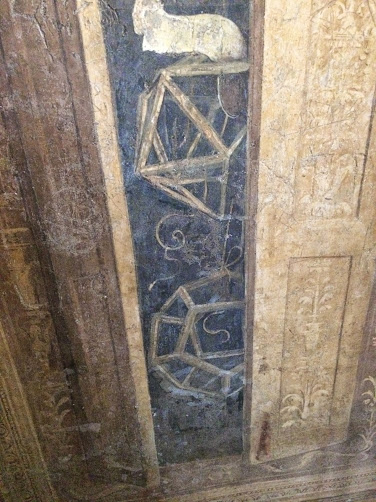/ MATHEMATICS, ART, GEOMETRY

# Platonic Solids and the School of Athens

## Euclid in The School of AthensThis is a very special fresco painting by Italian Renaissance artist Raphael in the Vatican Museum called The School of Athens. It depicts the great philosophers of ancient Greece, famously with Plato pointing up and Aristotle pointing down.Just to the right of me you can see Euclid doing some geometry on a tablet. Euclid’s contribution to mathematics is of course well known. The significance of his surviving masterpiece The Elements cannot be overstated, though the results and proofs collected in The Elements are not due entirely (or even mostly) to Euclid himself. One of the major results in The Elements is the construction of the five so-called Platonic solids and the proof that there are only five such solids. In fact, some commentators suggest that the establishment of this fact is the primary motivation of The Elements.

A platonic solid is a solid having faces all of which are the same regular polygon and with the same number of faces meeting at each vertex. If $p$ is the number of edges of each face and $q$ is the number of faces at each vertex, then each solid is uniquely identified by the ordered pair ${p, q}$ (called the Schläfli symbol). Here they all are:

Table Source: Platonic Solid on Wikipedia.

## Symmetry

Each platonic solid has a high degree of symmetry. By rotating a given solid along various axes, one can obtain exactly the same solid in the same orientation. These rotation operations under which the solid is invariant form a special kind of algebraic group called a symmetric group.

The dual of a regular polyhedron having $p$ vertices and $q$ faces is the solid having $q$ vertices and $p$ faces–one simply interchanges the roles of the vertices and edges to obtain the dual. Notice that the dual of each platonic solid is another platonic solid. This is such a satisfying fact to me.

## Leaving the School of Athens

Of course there are many other interesting facts about the platonic solids. For example, the Golden Ratio $\phi:=\frac{1+\sqrt{5}}{2}$ is related to the platonic solids. You will have to check out Wikipedia for the details.

That the ancients proved there are only five platonic solids does not diminish how mathematically interesting this fact is to me. A natural question to ask is, how many platonic solids (that is, platonic polytopes) are there in four dimensions? It turns out there are six. (One of them is trivial to find: Just add another point to the tetrahedron to form a 4-simplex.) But what is really interesting is that in every dimension greater than four there are only three platonic solids. So what is special about dimensions three and four?As I walked toward the exit of the room with The School of Athens fresco I found a painting of two platonic skeletons in a closet. It is fitting to find these geometric objects so close by the ancient Greek’s who studied their mathematical properties so extensively.#### Robert Jacobson

R&D-oriented computer scientist, mathematician, and software engineer with broad experience. I have particular interests in compilers, programming languages, and virtual machines; computer vision and machine learning; and algorithm design and mathematical programming.